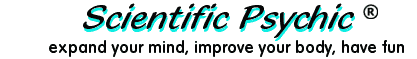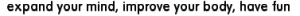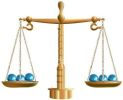Index# The Odd Ball Problem

There are twelve identical-looking balls, but one is either heavier or lighter than the other eleven. How can you determine which is the odd ball and find out whether this ball is heavier or lighter than the others using only three weighings with a balance?Label the balls from 1 to 12 to identify them.
Weigh 1, 2, 3, 4 against 5, 6, 7, 8:
1. If they balance, 9, 10, 11, 12 contain the odd ball.
Weigh 6, 7, 8 against 9, 10, 11.
1. If they balance, 12 is the odd ball. Weigh 12 against any other ball to discover whether it is heavy or light.
2. If 9, 10, 11 are heavy, they contain an odd heavy ball. Weigh 9 against 10. If they balance, 11 is the odd heavy ball, otherwise the heavier of 9 and 10 is the odd ball.
3. If 9, 10, 11 are light, we use the same procedure to reach the same conclusion for the odd light ball.

2. If 5, 6, 7, 8 are heavy, either they contain an odd heavy ball or 1, 2, 3, 4 contain an odd light ball.
Weigh 1, 2, 5 against 3, 6, 10.
1. If they balance, the odd ball is 4 (light) or 7 or 8 (heavy). Weigh 7 against 8. If they balance 4 is light, otherwise the heavier of 7 and 8 is the odd heavy ball.
2. If 3, 6, 10 are heavy, the odd ball can be 6 (heavy) or 1 or 2 (light). Weigh 1 against 2. If they balance 6 is heavy, otherwise the lighter of 1 and 2 is the odd light ball.
3. If 3, 6, 10 are light, the odd ball is 3 and light or 5 and heavy. We thus weigh 3 against 10. If they balance, 5 is heavy, otherwise 3 is light.

3. If 5, 6, 7, 8 are light we use a similar procedure to that in II.